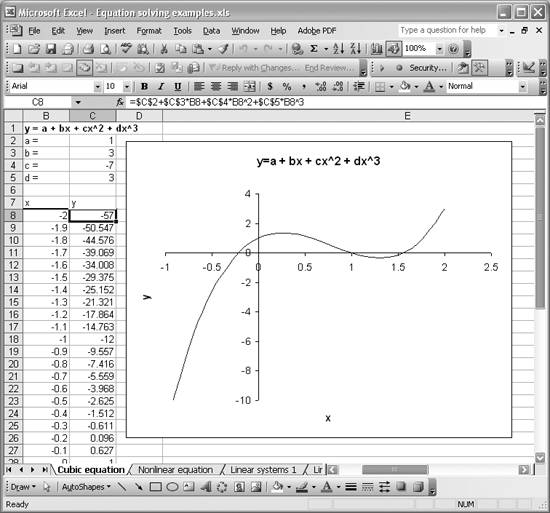# Finding Roots Graphically

Problem

You want to find the roots of an equation graphically.

Solution

Write the equation in the form y = g(x), compute values for y for a given range of x-values, and plot the results using Excel's charting features. Refer to Chapter 4 for more information on charting in Excel.

Discussion

When faced with finding roots to an equation that you can't solve by hand, the first thing I recommend you do is chart it. This will give you valuable insight into the nature of the equation and where its roots lie. If you can write the equation in the form of y = g(x), where x is the independent variable and y the dependent variable, then you can easily set up a few columns computing y for various values of x.

This may all seem trivial and frankly, in terms of spreadsheet operations, it is very simple to perform. However, when dealing with a particularly ugly equation with potentially multiple roots, you'd better have a good idea of where those roots lie. This is because most iterative methods for solving equations require an initial guess as to where the roots lie. Some methods require two initial guesses, while others require you to bracket the roots. The success of these various methods for finding roots lies in the quality of your initial guess. (See the other recipes in this chapter for specific examples.)

Consider the third-order polynomial equation:This equation has, of course, three roots. That is, there are three values of x that yield a y-value of zero. This is readily confirmed by plotting the curve for y versus x in Excel. Figure 9-6 shows how this particular equation looks when plotted in Excel.

Figure 9-6. Third-order polynomial plotAs you can see, there are indeed three zero crossings; that is, there are three values of x that yield zero for y. To plot this curve, I set up two columns: one containing given values for x and the other containing calculated values for y. These are shown in columns B and C, respectively, in Figure 9-6. I assumed arbitrary coefficients for this example and placed them in cells C2 through C5. The formula bar shows how I constructed the formula for computing y-values corresponding to each x. (Cell C8 is selected in the screenshot, so you see its formula in the formula bar.) The formula is of the form =\$C\$2+\$C\$3*B8+\$C\$4*B8^2+\$C\$5*B8^3.

At this point, you have a clear picture of how this equation behaves and where its roots lie. You have a few options now to actually find the particular values for x that yield y = 0. You can approximate the xs by interpolating between the tabulated values contained in columns B and C. Essentially you look for the xs corresponding to where the y-values go from negative to positive and positive to negative and then interpolate between those tabulated values. Alternatively, you can use iterative techniques to find the x-values. Such techniques are illustrated in Recipes 9.2 and 9.3. Recipe 9.6 shows how to find the roots of the cubic equation discussed here, using Newton's method and the secant method.

Now, let me ask you what happens when you're dealing with an equation that you can't write in the form y = g(x). Well, that presents a problem when you attempt to plot it using the straightforward approach discussed in this recipe. Fortunately, Excel offers other tools that you can leverage to get around such a difficulty. Check out Recipe 9.2 to see what I mean.Excel Scientific and Engineering Cookbook (Cookbooks (OReilly))
ISBN: 0596008791
EAN: 2147483647
Year: N/A
Pages: 206
Authors: David M Bourg